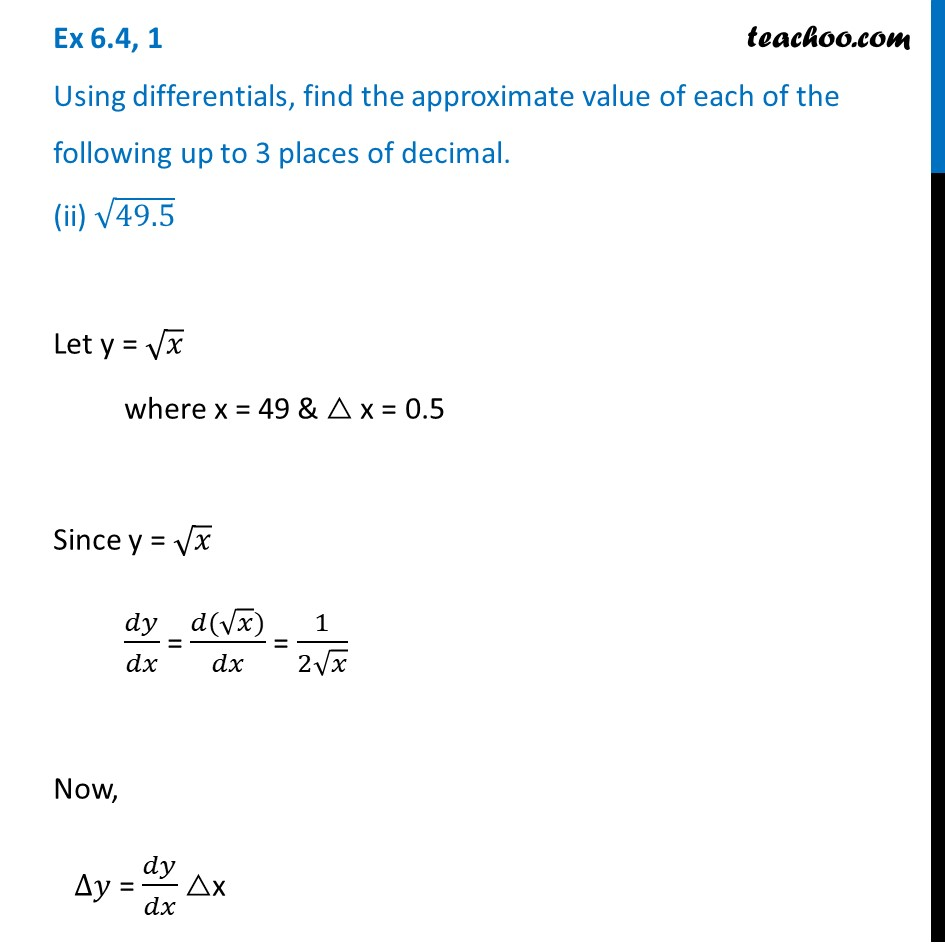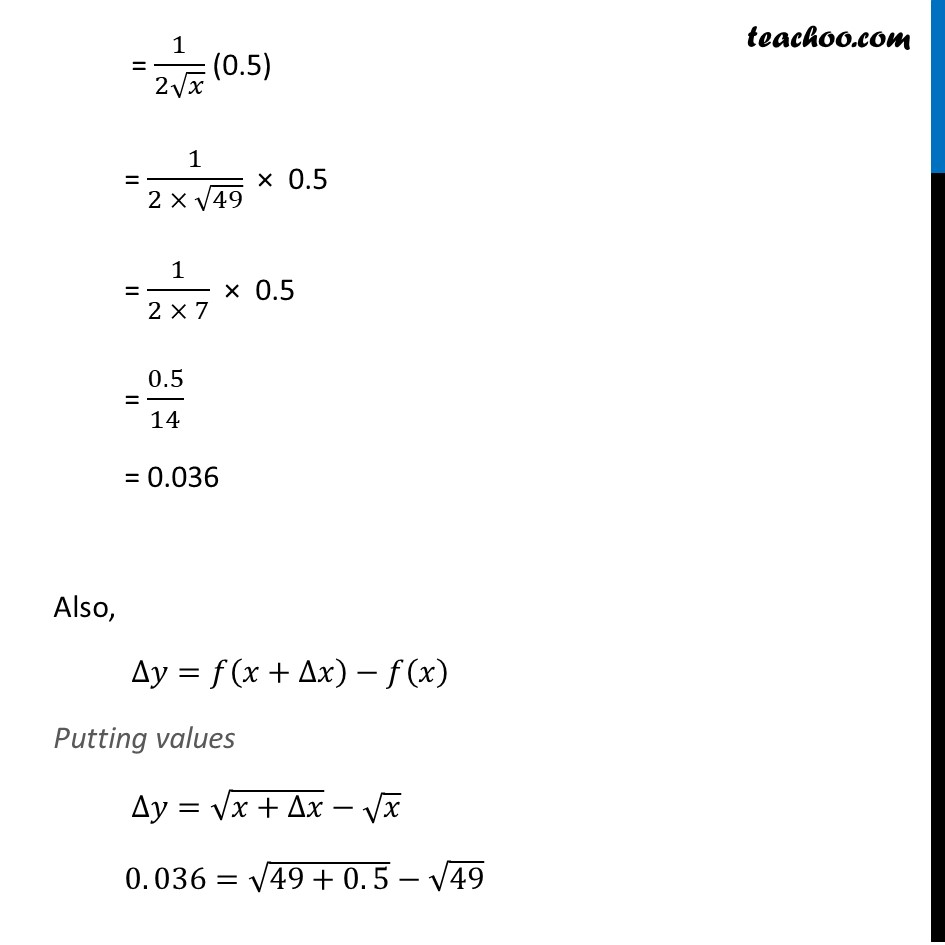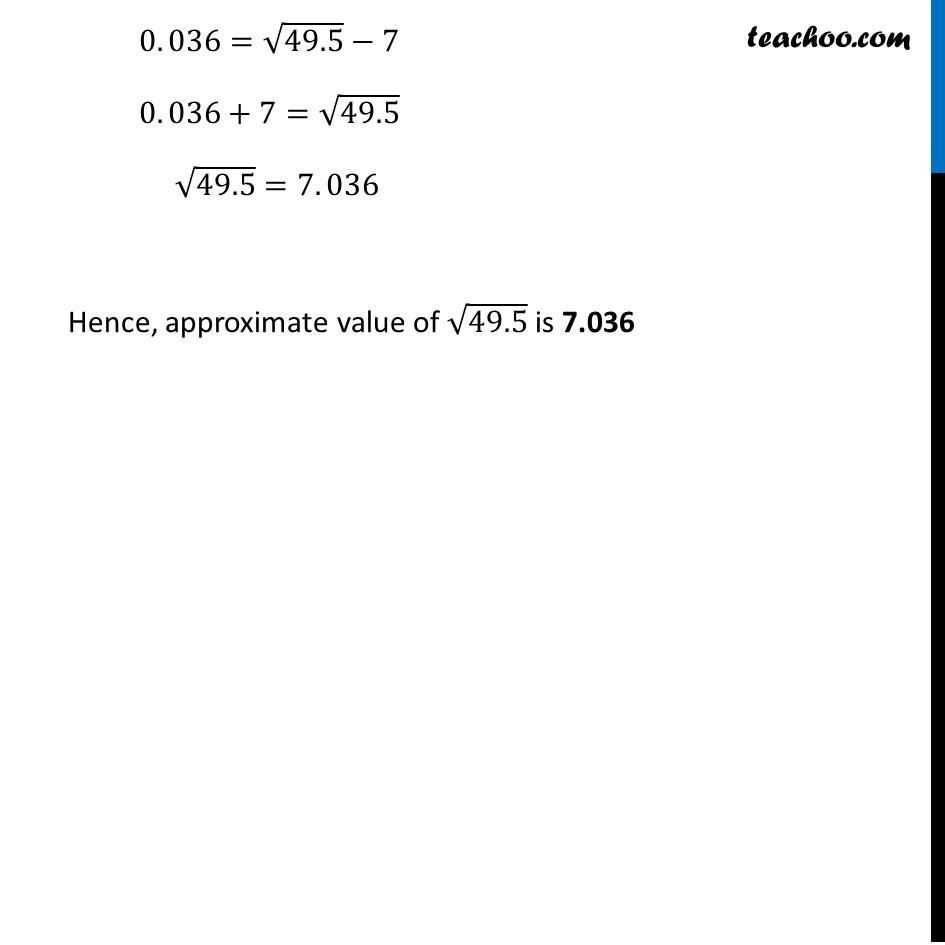Approximations (using Differentiation)

Chapter 6 Class 12 Application of Derivatives
Serial order wiseLearn in your speed, with individual attention - Teachoo Maths 1-on-1 Class

### Transcript

Question 1 Using differentials, find the approximate value of each of the following up to 3 places of decimal. (ii) √49.5Let y = √𝑥 where x = 49 & △ x = 0.5 Since y = √𝑥 𝑑𝑦/𝑑𝑥 = (𝑑(√𝑥))/𝑑𝑥 = 1/(2√𝑥) Now, ∆𝑦 = 𝑑𝑦/𝑑𝑥 △x = 1/(2√𝑥) (0.5) = 1/(2 × √49) × 0.5 = 1/(2 × 7) × 0.5 = 0.5/14 = 0.036 Also, ∆𝑦=𝑓(𝑥+∆𝑥)−𝑓(𝑥) Putting values ∆𝑦=√(𝑥+∆𝑥)−√𝑥 0. 036=√(49+0. 5)−√49 0. 036=√49.5−7 0. 036+7=√49.5 √49.5=7. 036 Hence, approximate value of √49.5 is 7.036